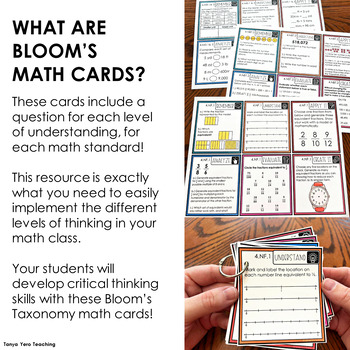# Bloom's Taxonomy 4th Grade Math Question Cards ALL STANDARDS 170 CARDS!Subject
Resource Type
Format
PDF (2 MB|32 pages)
Standards
\$8.00
List Price:
\$10.00
You Save:
\$2.00
\$8.00
List Price:
\$10.00
You Save:
\$2.00

### Description

This purchases include 170 Bloom's Taxonomy question cards!

What are Bloom’s Taxonomy Math Cards?

Bloom’s Taxonomy question cards are designed for teachers to use in their math classroom to ensure that they are targeting the different levels of questioning. Teaching math is a complex process that requires a lot of moving parts. These question cards will make your life easier with their ready to use formatting and color coded template. There are 6 cards for each 4th grade math standard.

How can I use these cards?

You can use these in any way! They can be used during your whole group or small group instruction and intervention/enrichment time. They can even be given to your students as task cards during rotations!

How do I store the Cards?

You can simply print the pages out and laminate them. They can be kept in a binder or folder to grab during your math instruction. They can also be cut out, hole-punched and placed on a binger ring for easy use.

Standards & Topics Covered

Number and Operation in Base Ten

➥ 4.NB.1 - Place Value Concepts

➥ 4.NBT.2 - Number Form, Word Form, Expanded Form, and Comparing of whole numbers

➥ 4.NBT.3 - Rounding multi-digit whole numbers

➥ 4.NBT.4 - Adding & Subtracting Whole Numbers

➥ 4.NBT.5 - Multiplying whole numbers

➥ 4.NBT.6 - Dividing whole numbers

Operations & Algebraic Thinking

➥ 4.OA.1 - Interpreting multiplication equations

➥ 4.OA.2 - Multiplying and dividing word problems

➥ 4.OA.3 - Solving multistep word problems, including interpreting remainders

➥ 4.OA.4 - Factors and multiples, Identifying prime and composite numbers within 100

Number and Operation - Fractions

➥ 4.NF.1 - Equivalent fractions

➥ 4.NF.2 - Comparing fractions

➥ 4.NF.3 - Adding and subtracting fractions with like denominators, decomposing fractions, adding and subtracting mixed numbers with like denominators

➥ 4.NF. 4 - Multiplying a fraction by a whole number

➥ 4.NF.5 - Adding and subtracting fractions with denominators of 10 and 100

➥ 4.NF.6 - Decimal notation for fractions with denominators of 10 and 100

➥ 4.NF.7 - Comparing decimals to hundredths

Measurement and Data

➥ 4.MD.1 - Measurement and converting measurement with the customary and metric systems of length, weight, mass, liquid volume, and time

➥ 4.MD.2 - Solving measurement word problems

➥ 4.MD.3 - Area and perimeter of rectangles

➥ 4.MD.4 - Line plots

➥ 4.MD.5 - Angles within a circle

➥ 4.MD.6 - Measuring angles with a protractor

➥ 4.MD.7 - Additive angle measurement, decomposing angles

Geometry

➥ 4.G.1 - Identifying points, lines, line segments, rays, angles, perpendicular and parallel lines in 2D shapes

➥ 4.G.2 - Classifying 2D figures, types of triangles

➥ 4.G.3 - Symmetry

➥ 4.NBT.4 - Adding & Subtracting Whole Numbers

➥ 4.NBT.5 - Multiplying whole numbers

➥ 4.NBT.6 - Dividing whole numbers

Total Pages
32 pages
Included
Teaching Duration
1 Year
Report this Resource to TpT
Reported resources will be reviewed by our team. Report this resource to let us know if this resource violates TpT’s content guidelines.

### Standards

to see state-specific standards (only available in the US).
Compare two decimals to hundredths by reasoning about their size. Recognize that comparisons are valid only when the two decimals refer to the same whole. Record the results of comparisons with the symbols >, =, or <, and justify the conclusions, e.g., by using a visual model.
Use decimal notation for fractions with denominators 10 or 100. For example, rewrite 0.62 as 62/100; describe a length as 0.62 meters; locate 0.62 on a number line diagram.
Express a fraction with denominator 10 as an equivalent fraction with denominator 100, and use this technique to add two fractions with respective denominators 10 and 100. For example, express 3/10 as 30/100, and add 3/10 + 4/100 = 34/100.
Recognize a line of symmetry for a two-dimensional figure as a line across the figure such that the figure can be folded along the line into matching parts. Identify line-symmetric figures and draw lines of symmetry.
Classify two-dimensional figures based on the presence or absence of parallel or perpendicular lines, or the presence or absence of angles of a specified size. Recognize right triangles as a category, and identify right triangles.